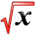# OptimizationThis article is part of the MathHelp Tutoring Wiki

## Optimization

Lecture notes coming soon...

• Q** Your room has a window whose height is 1.5 meters.. The bottom edge of the window is 10 cm above your eye level. How far away from the window should you stand to get the best view? ("Best view" means the largest visual angle, ie. angle between the lines of sight to the bottom and to the top of the window.)
• A** First we formulate a couple relationships: tan(alpha) = 0.1/x, and tan(alpha + theta) = 1.6/x. Then we use the multiple angle formula for tangent to make it tan(alpha + theta) = tan(alpha) + tan(theta)/ (1 - tan(alpha)tan(theta) ) = 1.6/x. Simplifying a bit, and sub in tan(alpha) = 0.1/x to get tan(theta) = 1.5x/(x^2 + 0.16). Because tan(theta) is a function of x, we define f(x) = tan(theta) = 1.5x/(x^2 + 0.16). As we know that tan(theta) is an increasing function of theta, so maximizing theta is the same as maximizing tan(theta) (THIS IS THE KEY INSIGHT TO SOLVING THIS QUESTION). Therefore all we need to do is to maximize tan(theta), or equivalently, maximize f(x). To maximize, we differentiate f'(x) = (-1.5x^2 + 0.24)/(x^2 + 0.16)^2 = 0, and solving yields x = 0.4.

Note: another method is to write theta = arctan(1.6/x) - arctan(0.1/x), and then use derivative formulas for arctan to differentiate.

• Q** Jack and Jill have an on-again off-again love affair. The sum of their love for one another is given by the function

y( t) = sin(2t) + cos(2t).

( a) Find the times when their total love is at a maximum.

(b ) Find the times when they dislike each other the most.

• A** We need to find the max and the min, so we differentiate. y' = 2cos(2t) - 2sin(2t) = 0. To solve this, we have cos(2t) = sin(2t). We need to find the angles (2t) where sine and cosine are the same. From high school (if you don't remember this, you have to review), sine and cosine are equal at angles pi/4 and 5pi/4. However, because the function oscillates, we have to find the general representation of the solution, which is pi/4 + 2n*pi, and 5pi/4 + 2n*pi, for any integer n. The reason we add this 2n*pi term is because the period of trig functions are 2pi. So we have 2t = pi/4 + 2n*pi and 5pi/4 + 2n*pi. Then, t = pi/8 + n*pi and 5pi/8 + n*pi. Checking second derivative to see that t = pi/8 + n*pi is the max and t = 5pi/8 + n*pi is the min.
• Q** A farmer wants to construct a fenced enclosure for his Alpacas. one side of the enclosure will be his existing farm house. Assuming that a rectangular geometry, what is the largest possible area for the enclosure if he only has 100 meter of fencing?
• A**we may first draw what the fencing will be like to help us understand the question.

We assume two sides of the fencing is x meter and y meter respectively. so we can write the Area as

```A= x*y
```

and from the question, we know that

2y+x=100

so now we can rewrite Area so that there's only one variable y

A=x*y=(100-2y)*y=100y-2y^2 (0<=y<=50)

Let's find the global maximum of A on this interval

First we find the critical points, so when the first derivative of A is equal to 0

A'=100-4y

So A'=0, y=25

Let's compute the area A, at the possible maxima.

y=0, A=0

y=25,A=1250

y=50,A=0

So we can see that when y=25, the area A, is maximized

At y=25, we find x=100-2y=50

Thus, the alpacas will be most comfortable in an enclosure that is 25 meter by 50 meter.

• Q** An open box is formed from a square sheet of cardboard by cutting equal squares from each corner and folding up the edges. If the dimensions of the cardboard are 18cm by 18cm, What should be the dimensions of the box so as to maximize the volume, and what is the maximum volume?
• A**

Area of the box = Area of the cardboard sheet => Area of the box = 18*18 = 324cm^2 Volume of the box = height * side ^2 (since sides are equal) = h*s^2 also 2*h + s = 18 => s = 18 - 2*h

V = h*(18-2h)^2

dV/dh = (18-2h)^2 + 2*h*(-2)*(18-2h) = 0

18-2h-4h=0 => h=3cm => s=12cm => V=432cm^3